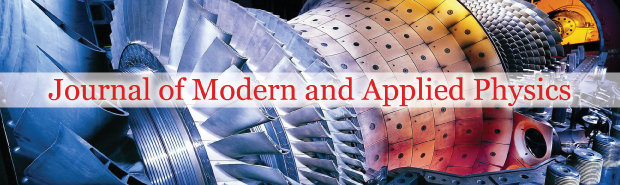All submissions of the EM system will be redirected to Online Manuscript Submission System. Authors are requested to submit articles directly to Online Manuscript Submission System of respective journal.### The electrical nature of gravity

Author(s): Scott Sibley*

Gravity was established as electrical in nature because the unit electrical charge calculated for gravitation based on measured G (1.4396077 ×10-34 C), adjusted for composition, agrees nearly perfectly (within 0.014%) with the calculated gravitational charge (1.4399644 × 10-34 C), based on the square of the velocity of light, where c2=
(8.8.9875518 x 10-16 m2/s2× primary electrical charge (1.602176487 ×10-19 C), the product of which is entirely electrical. c2× 10-32 m2/s2 was calculated and derived, a priori, as the ratio of secondary to primary electrical charge (e2/e1). Gravitational charge (e2), a new constant (1.4399644×10-34 C), was calculated in Coulombs to 8 significant figures. G was calculated and derived to within 0.05% of its measured value, using c2 ; primary charge; the electrical constant; the magnetic constant; and the weighted average mass number to atomic weight ratio of the Milky Way Galaxy. The composition dependence of G was established. G, with new units, were restored as: G=6.6775860 × 10-11 NN × (C2)(s2)(m-2). Gravity is a probable source of electromagnetism.Gravity was calculated to be net attractive. The variability of G in the Milky Way Galaxy is explained.

Full-Text | PDF

###### Journal of Modern and Applied Physics peer review process verified at publonsTop